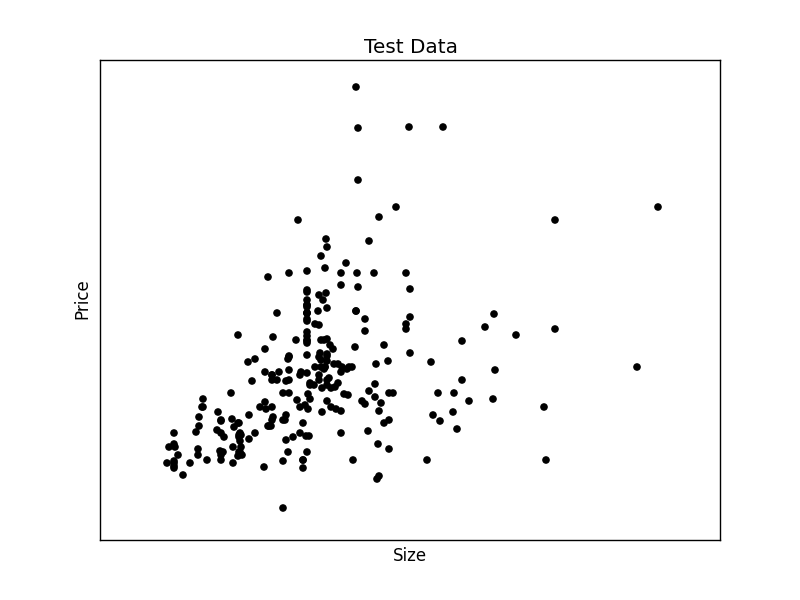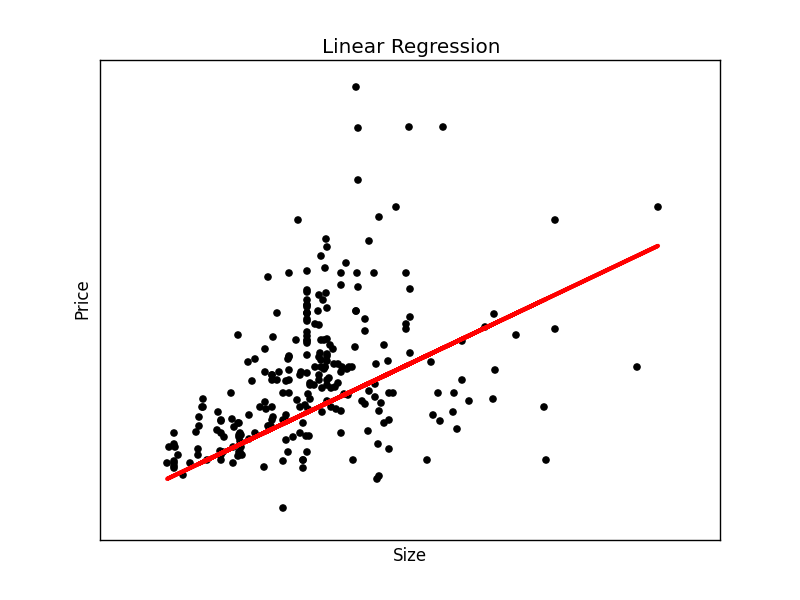# Linear Regression

How does regression relate to machine learning?

Given data, we can try to find the best fit line. After we discover the best fit line, we can use it to make predictions.

Consider we have data about houses: price, size, driveway and so on. You can download the dataset for this article here.

Data can be any data saved from Excel into a csv format, we will use Python Pandas to load the data.

Related courses

Required modules
You shoud have a few modules installed:

Load dataset and plot
You can choose the graphical toolkit, this line is optional:

We start by loading the modules, and the dataset. Without data we can’t make good predictions.

The first step is to load the dataset. The data will be loaded using Python Pandas, a data analysis module. It will be loaded into a structure known as a Panda Data Frame, which allows for each manipulation of the rows and columns.

We create two arrays: X (size) and Y (price). Intuitively we’d expect to find some correlation between price and size.

The data will be split into a trainining and test set. Once we have the test data, we can find a best fit line and make predictions.

Finally we plot the test data.We have created the two datasets and have the test data on the screen. We can continue to create the best fit line:

This will output the best fit line for the given test data.To make an individual prediction using the linear regression model:

1.